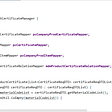# JS |Minimum swaps to get together 1’s

## Amazon Interview Question

`Input : arr[] = [ 1, 0, 1, 0, 1 ]Output : 1Explanation: Only 1 swap is required to group all 1's together. Swapping index 1and 4 will give arr[] = [1, 1, 1, 0, 0]Input : arr[] = [1,0,1,0,1,0,0,1,1,0,1]Output : 3 Swaps required`

Efficient approach, using the concept of window-sliding technique(please go through this once)

• First count total number of 1’s in the array
• Suppose this count is x, now find the subarray of length x of this array with a maximum number of 1’s using the concept of window-sliding
• Maintain a variable to find the number of 1’s present in a subarray in O(1) extra space and for each sub-array maintain maxOnes Variable and at last Return numberOfZeros (numberOfZeroes = x — maxOnes)

Time Complexity: O(n)
Auxiliary Space: O(1)

`function minSwaps(arr, n) {let numberOfOnes = 0;// find total number of all 1's in the arrayfor (let i = 0; i < n; i++) {  if (arr[i] == 1) numberOfOnes++;}let x = numberOfOnes;let count_ones = 0, maxOnes;// Find 1's for first subarray of length xfor(let i = 0; i < x; i++){   if(arr[i] == 1) count_ones++;}maxOnes = count_ones;/** using sliding window technique to find max number of ones in subarray of length x **/for (let i = 1; i <= n-x; i++) {/** first remove leading element and check if it is equal to 1 then decrement the value of count_ones by 1 **/   if (arr[i - 1] == 1)     count_ones--;/** Now add trailing element and check if it is equal to 1 Then increment the value of count_ones by 1 **/   if(arr[i + x - 1] == 1)     count_ones++;   if (maxOnes < count_ones)     maxOnes = count_ones;   }/ ** calculate number of zeros in subarray of length x with maximum number of 1’s **/   let numberOfZeroes = x - maxOnes;return numberOfZeroes;}`

Don’t forget to follow The Lean Programmer Publication for more such articles, and subscribe to our newsletter tinyletter.com/TheLeanProgrammer

--

--

--

## More from TheLeanProgrammer

The biggest power in the world is to be able to give life to something, and guess what? Code gives you this ability! Here in this publication, we build stuff, we share knowledge in tech, and share our stories, feel free to join — https://theleanprogrammer.com/writer-request/

## Arrays → In → Java## Recapitulation of BabySunCoin PROJECT AMA event held at AMA LOVERS CLUB.## Minimum Window Subsequence## Localization Changes in Java 9## Renovate: Dependency Management## Is GNOME Theming Dead ..or Not?## How to implement flow coordinator pattern## Use of @Async asynchronous method + @Transactional transaction processing## iAmSonika | www.startlearncoding.com

Working in Walmart as UI Developer | Mentor | React | JavaScript | HTML | CSS

## keep single copy of node_modules for multiple projects## Understanding the work loop in React